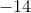# AP Computer Science A : Evaluating Numerical Expressions

## Example Questions

### Example Question #1 : Evaluating Numerical Expressions

Consider the following code:

int i = 85, j = 2, k = 4;

i--;

j++;

k -= 6;

i = i / j + i / k;

What is the value of i in the code above?Explanation:

It is probably easiest to give a running commentary on the code in order to understand it. See the comments in bold below:

int i = 85, j = 2, k = 4;

i--; // After this, i = 84

j++; // After this, j = 3

k -= 6; // After this, k = k - 6 or k = -2

/*

Consider this in several steps:

i / j = 84 / 3 = 28

i / k = 84 / -2 = -42

Thus, the final expression is:

i = 28 - 42 = -14

*/

i = i / j + i / k;

### Example Question #2 : Evaluating Numerical Expressions

Consider the code below:

int a = 24, b = 17, c = 5, d = 7;

b = b % d + a / b;

a %= b;

a -= 12;

What is the value of a at the end of the code above?Explanation:

The easiest way to trace code like this is to provide running commentary in bold comments. See these below:

int a = 24, b = 17, c = 5, d = 7;

/*

The modulus (%) is the remainder of a division. So . . .

b % d = 17 % 7 = 3 (17 divided by 7 is 2 remainder 3.)

a / b = 1 (Remember, integer division truncates the decimal portion.)

Thus, we have:

b = 3 + 1 = 4

*/

b = b % d + a / b;

a %= b; // This is the same as a = a % d, which is a = 24 % 4 =  0 (No remainder)

a -= 12; // Remember that this is the same as a = a - 12, which is a = 0-12 or -12.

### Example Question #3 : Evaluating Numerical Expressions

What is the Unicode value for the letter "W"?

U+0087

U+007C

U+005C

U+0057

U+0047

U+0057

Explanation:

The Unicode Standard is Java's attempt to simplify the translation of characters and symbols to input the computer can understand, while being able to work on all Java machines. The first 128 numbers (0-127, 0x0000-0x007F) are for basic Latin. Under this character encoding scheme, the letter 'W' is denoted by U+0057.

The standard for writing Unicode values is U+####, where the number is in hexadecimal format, not a base 10 number.

### Example Question #4 : Evaluating Numerical Expressions

#include <iostream>

using namespace std;

int main()

{

bool bin=true;

int var=bin*2 +1;

int triple=5;

int final= (bin&var) &triple;

cout<<final;

return 0;

}

What is the output of this code?

3

2

1

0

false

1

Explanation:

Taking a look at the first line in main, the variable bin is defined as true, which we know to be 1. We see in the next line that the variable var is 2 * bin +1. The trick here is that you can actually do math with boolean values, so the value of var is 3.

The value of triple is 5.

The the variable final asks us to AND bin and var first, then AND triple.

To do this, we can convert all three numbers into binary notation to make it easier.

1 in binary is 001.

3 in binary is 011.

5 in binary is 101.

1&3 =1.

1&5=1.

### Example Question #1 : Evaluating Numerical Expressions

int x = 2893;

1. What does the expression " x / 10 " evaluate to?
2. What does the expression " x % 10 " evaluate to?

1. 289.3
2. 3
1. 289
2. 3
1. 289.3
2. 893
1. 289
2. 2000
1. 3
2. 289
1. 289
2. 3
Explanation:
1. 2893 / 10 = 289, since x is an int, it does not store the decimal value

2. 2893 % 10 = 3 because the remainder of 2893/10 is 3

### Example Question #2 : Evaluating Numerical Expressions

int a;

a= 1+1 * 2 + 4 / 5;

System.out.println(a);

What would the code output?

2.4

0

3.8

3

2